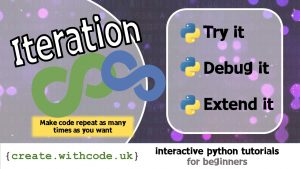## The Theory:Iteration in python: Make code repeat as many times as you want

There are two types of iteration: definite iteration and indefinite iteration.

Both definite and indefinite iteration involve repeating a section of code multiple times.

Definite iteration is when you know exactly how many times your code will repeat (e.g. a `for` loop)

Indefinite iteration is when you don’t know exactly how many times your code will repeat: it might repeat forever (e.g. a `while` loop)

#### Definite iteration

Definite iteration is useful when you want to repeat a section of code a specific number of times, for example, performing the same operation on each item in a list.

In Python, you can use a `for` loop to iterate (repeat) through a list of values.

The code above uses the variable `i` to iterate through each value in the range from 0 to 9. This works as a loop. The first time the loop repeats `i` is set to 0. The next time `i` is set to 1 and this keeps repeating until `i` is set to 9.

The code above uses the variable `a` to iterate through each value in the list of `animals`. The code that is indented repeats once for each value of `animals` using `a` to store the current value.

Definite iteration is often called count-controlled iteration because the number of times the code repeats is controlled by a number.

#### Indefinite iteration

Indefinite iteration is often called condition-controlled iteration because the number of times the code repeats is controlled by a boolean expression

A boolean expression is a value or combination of values / variables / functions which evaluate to an answer of either True or False

For example:

The example above repeats while the `count_down` variable stores a value which is greater than 0. The boolean expression is `count_down > 0` which is either `True` or `False` depending on the value of `count_down`.

Sometimes it’s useful to repeat until a condition is met. The example below will repeat until the user types in “yes” to the irritating car journey question of “Are we nearly there yet?”

Page 1: Intro

Page 2: The theory: learn what you need to know as fast as possible.

Page 3: Try it: try out and adapt some working python code snippets.

Page 4: Debug it: Learn how to find and fix common mistakes.

Page 5: Extend it: Choose a project idea to use your newfound python skills.